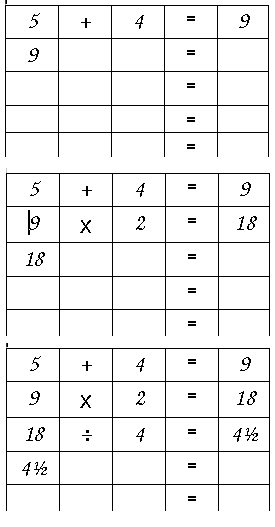# Arithmetic Math Game

### Here's a math game to make that helps kids enjoy practicing their arithmetic

By

I was wondering how I could make a good arithmetic math game for kids. A game that would make arithmetic fun - a competitive game. A math game with elements of strategy, luck, and good old fun. A few ideas ran through my head, until suddenly - aha!

If what I've described is what you are looking for in an arithmetic math game, well your search is over. Read on, and I'll explain :

• The playing pieces of this game, and how to make them,
• How to avoid making them if you've no patience with glue and scissors,
• The rules, with variations for different age groups.

Ok, the pieces first. If you download and print this file, you'll then have

• Some dice to cut out and make,
• Some numbered cards to cut out, and
• Some scoresheets to photocopy.

It's best to print everything onto cardboard. To make the dice, first press along each fold with a blunt knife, then fold and glue the tabs to the inside of the faces, so the printed symbols face out.

This will be troublesome for some people - especially if you are a teacher and you want to get your whole class playing this game. Here's some tips to make life easier.

• Notice that two of the dice are ordinary dice. If you happen to have some dice handy, you don't need to cut and fold the blue or yellow dice.
• In fact, you don't need to cut out the third die either. Instead, get a die which is a different color or size from the first two, and use this table to convert numbers to symbols :
2. subtract
3. multiply
4. divide
6. wild (any operation)

Once you have the different playing pieces ready, start the arithmetic math game as follows.

• Make sure each player has a gamesheet, and a pencil or pen.
• Mix up the cards in a bag or shoebox or similar. Then each player draws one card, and lays it on the table. This card shows their goal number.
• Each player rolls a single ordinary die. The number shown is their starting number. The player should write the starting number in the top left corner of their gamesheet.
• The player with the largest goal number goes first.

The players then take turns, in an anticlockwise direction, until there is a winner. On each turn :

• The player rolls the three dice. This will show an arithmetical operation (either addition, subtraction, multiplication or division) and two numbers (each between one and six).
• There are two special symbols on the operation die.
• If it shows the "plus-or-minus" symbol, the player can choose to use either plus or minus.
• If it shows a question mark, the player can use any of the four operations plus, minus, times and divide.
• (Optional Rule) If the player is not satisfied with the numbers and symbols showing, he or she may pick up the dice and roll again (once only).
• The player writes the operation in the second column of their gamesheet.
• The player chooses one of the two numbers, and writes this in the third column of the worksheet.
• Then, the player works out the result of the arithmetic problem shown, and writes the answer in the fifth column of the worksheet (after the equals sign).
• If the answer is the player's goal number, the player has won! Otherwise, the answer should be copied into the first column of the next row. Then, it will be the next player's turn.The pictures at the right show what a player's game worksheet might look as a game progresses. Suppose their starting number was 5.

• On the player's first roll, they get a +, a 6 and a 4. The player chooses 4, so (given the starting number 5) the first row shows 5 + 4 = 9.
• On the second roll, the player is lucky enough to get a ? on the operations die. The other dice show a 2 and a 6. Here, the player chooses the number 2, and for their operation, they can choose anything. They chose multiplication.
• On the third roll, the numbers were 4 and 5, but the operation die showed division! Ouch!

For different age groups, the arithmetic math game might be too easy, or too hard. Here's some suggestions you might like to try.

• To make the game easier
• Instead of two dice with numbers, use three. This will give more choice.
• If your class or children don't know fractions yet, there may be problems sometimes when the division operator comes up. You could allow a free re-roll if they can't avoid fractions.
• You could allow the use of a calculator
• Each player could choose more than one goal card, and they win if they reach any one of the goals.
• To make it harder
• Instead of getting to choose which of the two numbered dice the player can use, the number they use must be the sum of the two dice. In this case, you will have to allow re-rolls. Without any choice to make, the game would become a long frustrating game of chance. It would be better just to give a multiplication worksheet.
• The players choose more than one goal card, and must reach the sum of the numbers drawn.

Yours, Dr Mike.